Updating search results...

# 91 Results

View
Selected filters:
• Statistics and Probability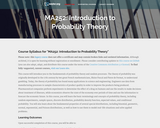Unrestricted Use
CC BY
Rating
0.0 stars

In this course, the student will learn the basic terminology and concepts of probability theory, including sample size, random experiments, outcome spaces, discrete distribution, probability density function, expected values, and conditional probability. The course also delves into the fundamental properties of several special distributions, including binomial, geometric, normal, exponential, and Poisson distributions. Upon successful completion of this course, the student will be able to: Define probability, outcome space, events, and probability functions; Use combinations to evaluate the probability of outcomes in coin-flipping experiments; Calculate the union of events and conditional probability; Apply Bayes's theorem to simple situations; Calculate the expected values of discrete and continuous distributions; Calculate the sums of random variables; Calculate cumulative distributions and marginal distributions; Evaluate random processes governed by binomial, multinomial, geometric, exponential, normal, and Poisson distributions; Define the law of large numbers and the central limit theorem. (Mathematics 252)

Subject:
Mathematics
Statistics and Probability
Material Type:
Full Course
Provider:
The Saylor Foundation
04/29/2019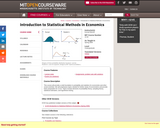Conditional Remix & Share Permitted
CC BY-NC-SA
Rating
0.0 stars

" This course will provide a solid foundation in probability and statistics for economists and other social scientists. We will emphasize topics needed for further study of econometrics and provide basic preparation for 14.32. Topics include elements of probability theory, sampling theory, statistical estimation, and hypothesis testing."

Subject:
Economics
Mathematics
Social Science
Statistics and Probability
Material Type:
Full Course
Provider:
M.I.T.
Provider Set:
M.I.T. OpenCourseWare
Author:
01/01/2009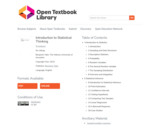Unrestricted Use
CC BY
Rating
0.0 stars

The target audience for this book is college students who are required to learn statistics, students with little background in mathematics and often no motivation to learn more. It is assumed that the students do have basic skills in using computers and have access to one. Moreover, it is assumed that the students are willing to actively follow the discussion in the text, to practice, and more importantly, to think.

Teaching statistics is a challenge. Teaching it to students who are required to learn the subject as part of their curriculum, is an art mastered by few. In the past I have tried to master this art and failed. In desperation, I wrote this book.

This book uses the basic structure of generic introduction to statistics course. However, in some ways I have chosen to diverge from the traditional approach. One divergence is the introduction of R as part of the learning process. Many have used statistical packages or spreadsheets as tools for teaching statistics. Others have used R in advanced courses. I am not aware of attempts to use R in introductory level courses. Indeed, mastering R requires much investment of time and energy that may be distracting and counterproductive for learning more fundamental issues. Yet, I believe that if one restricts the application of R to a limited number of commands, the benefits that R provides outweigh the difficulties that R engenders.

Another departure from the standard approach is the treatment of probability as part of the course. In this book I do not attempt to teach probability as a subject matter, but only specific elements of it which I feel are essential for understanding statistics. Hence, Kolmogorov’s Axioms are out as well as attempts to prove basic theorems and a Balls and Urns type of discussion. On the other hand, emphasis is given to the notion of a random variable and, in that context, the sample space.

I Introduction to Statistics
1 Introduction
2 Sampling and Data Structures
3 Descriptive Statistics
4 Probability
5 Random Variables
6 The Normal Random Variable
7 The Sampling Distribution
8 Overview and Integration
II Statistical Inference
9 Introduction to Statistical Inference
10 Point Estimation
11 Confidence Intervals
12 Testing Hypothesis
13 Comparing Two Samples
14 Linear Regression
15 A Bernoulli Response
16 Case Studies

Subject:
Mathematics
Statistics and Probability
Material Type:
Textbook
Author:
Benjamin Yakir
09/21/2021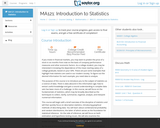Unrestricted Use
CC BY
Rating
0.0 stars

This course covers descriptive statistics, the foundation of statistics, probability and random distributions, and the relationships between various characteristics of data. Upon successful completion of the course, the student will be able to: Define the meaning of descriptive statistics and statistical inference; Distinguish between a population and a sample; Explain the purpose of measures of location, variability, and skewness; Calculate probabilities; Explain the difference between how probabilities are computed for discrete and continuous random variables; Recognize and understand discrete probability distribution functions, in general; Identify confidence intervals for means and proportions; Explain how the central limit theorem applies in inference; Calculate and interpret confidence intervals for one population average and one population proportion; Differentiate between Type I and Type II errors; Conduct and interpret hypothesis tests; Compute regression equations for data; Use regression equations to make predictions; Conduct and interpret ANOVA (Analysis of Variance). (Mathematics 121; See also: Biology 104, Computer Science 106, Economics 104, Psychology 201)

Subject:
Mathematics
Statistics and Probability
Material Type:
Full Course
Provider:
The Saylor Foundation
04/29/2019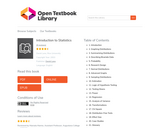Unrestricted Use
Public Domain
Rating
0.0 stars

Introduction to Statistics is a resource for learning and teaching introductory statistics. This work is in the public domain. Therefore, it can be copied and reproduced without limitation. However, we would appreciate a citation where possible. Please cite as: Online Statistics Education: A Multimedia Course of Study (http://onlinestatbook.com/). Project Leader: David M. Lane, Rice University. Instructor's manual, PowerPoint Slides, and additional questions are available.

1. Introduction
2. Graphing Distributions
3. Summarizing Distributions
4. Describing Bivariate Data
5. Probability
6. Research Design
7. Normal Distributions
9. Sampling Distributions
10. Estimation
11. Logic of Hypothesis Testing
12. Testing Means
13. Power
14. Regression
15. Analysis of Variance
16. Transformations
17. Chi Square
18. Distribution-Free Tests
19. Effect Size
20. Case Studies
21. Glossary

Subject:
Mathematics
Statistics and Probability
Material Type:
Textbook
Author:
06/25/2020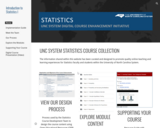Conditional Remix & Share Permitted
CC BY-NC-SA
Rating
0.0 stars

STUDENT LEARNING OUTCOMES (SLOS)
CO1 - Students should be able to describe important characteristics of a data set. (Describe can be in words or can mean to calculate values when appropriate)

CO2 - Students should be able to infer appropriate information from sample data. (verify, validate, conclude...to the population)

CO3 - Students should be able to interpret their results (from CO1 and CO2). (what does it mean? why is it useful?)

CO4 - Students should be able to communicate their results (from CO1, CO2, and CO3). (tell others, show others)

MODULES
Topic 1 - Sampling and Data

Topic 2 - Probability

Topic 3 - Probability and Sampling Distributions

Topic 4 - 1-Sample Confidence Intervals

Topic 5 - 1-Sample Hypothesis Testing

Topic 6 - 2-Sample Inference for Means

Topic 7 - 2-Sample Inference for Proportions

Topic 8 - Regression

Subject:
Mathematics
Statistics and Probability
Material Type:
Full Course
Module
Author:
University of North Carolina System
UNC System Digital Course Enhancement Initiative
04/16/2021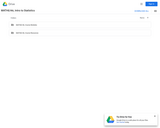Unrestricted Use
CC BY
Rating
0.0 stars

The main goal of the course is to highlight the general assumptions and methods that underlie all statistical analysis. The purpose is to get a good understanding of the scope, and the limitations of these methods. We also want to learn as much as possible about the assumptions behind the most common methods, in order to evaluate if they apply with reasonable accuracy to a given situation. Our goal is not so much learning bread and butter techniques: these are pre-programmed in widely available and used software, so much so that a mechanical acquisition of these techniques could be quickly done "on the job". What is more challenging is the evaluation of what the results of a statistical procedure really mean, how reliable they are in given circumstances, and what their limitations are.Login: guest_oclPassword: ocl

Subject:
Mathematics
Statistics and Probability
Material Type:
Full Course
Homework/Assignment
Lecture Notes
Syllabus
Provider:
Washington State Board for Community & Technical Colleges
Provider Set:
Open Course Library
10/31/2011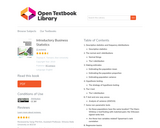Unrestricted Use
CC BY
Rating
0.0 stars

The book "Introductory Business Statistics" by Thomas K. Tiemann explores the basic ideas behind statistics, such as populations, samples, the difference between data and information, and most importantly sampling distributions. The author covers topics including descriptive statistics and frequency distributions, normal and t-distributions, hypothesis testing, t-tests, f-tests, analysis of variance, non-parametric tests, and regression basics. Using real-world examples throughout the text, the author hopes to help students understand how statistics works, not just how to "get the right number."

1. Descriptive statistics and frequency distributions

Descriptive statistics
2. The normal and t-distributions

Normal things
The t-distribution
3. Making estimates

Estimating the population mean
Estimating the population proportion
Estimating population variance
4. Hypothesis testing

The strategy of hypothesis testing
5. The t-test

The t-distribution
6. F-test and one-way anova

Analysis of variance (ANOVA)
7. Some non-parametric tests

Do these populations have the same location? The Mann-Whitney U testTesting with matched pairs: the Wilcoxon signed ranks test.
Are these two variables related? Spearman's rank correlation
8. Regression basics

What is regression?
Correlation and covariance
Covariance, correlation, and regression

Subject:
Mathematics
Statistics and Probability
Material Type:
Textbook
Author:
Thomas K. Tiemann
06/25/2020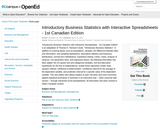Unrestricted Use
CC BY
Rating
0.0 stars

Chapter 1. Descriptive Statistics and Frequency Distributions
Chapter 2. The Normal and t-Distributions
Chapter 3. Making Estimates
Chapter 4. Hypothesis Testing
Chapter 5. The t-Test
Chapter 6. F-Test and One-Way ANOVA
Chapter 7. Some Non-Parametric Tests
Chapter 8. Regression Basics

Subject:
Mathematics
Statistics and Probability
Material Type:
Textbook
Provider:
BCcampus
Provider Set:
BCcampus Open Textbooks
Author:
Elon University
Thompson Rivers University; Thomas K. Tiemann
04/19/2016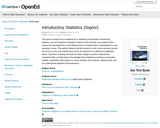Unrestricted Use
CC BY
Rating
0.0 stars

This book is meant to be a textbook for a standard one-semester introductory statistics course for general education students.Over time the core content of this course has developed into a well-defined body of material that is substantial for a one-semester course. The authors believe that the students in this course are best served by a focus on the core material and not by an exposure to a plethora of peripheral topics. Therefore in writing this book we have sought to present material that comprises fully a central body of knowledge that is defined according to convention, realistic expectation with respect to course duration and students maturity level, and our professional judgment and experience.

Subject:
Mathematics
Statistics and Probability
Material Type:
Textbook
Provider:
BCcampus
Provider Set:
BCcampus Faculty Reviewed Open Textbooks
Author:
Douglas S. Shafer
Zhiyi Zhang
10/28/2014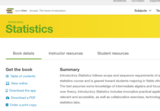Unrestricted Use
CC BY
Rating
0.0 stars

Introductory Statistics follows scope and sequence requirements of a one-semester introduction to statistics course and is geared toward students majoring in fields other than math or engineering. The text assumes some knowledge of intermediate algebra and focuses on statistics application over theory. Introductory Statistics includes innovative practical applications that make the text relevant and accessible, as well as collaborative exercises, technology integration problems, and statistics labs.

Access also available here: https://openstax.org/details/books/introductory-statistics

Sampling and Data
Descriptive Statistics
Probability Topics
Discrete Random Variables
Continuous Random Variables
The Normal Distribution
The Central Limit Theorem
Confidence Intervals
Hypothesis Testing with One Sample
Hypothesis Testing with Two Samples
The Chi-Square Distribution
Linear Regression and Correlation
F Distribution and One-Way ANOVA

Subject:
Mathematics
Statistics and Probability
Material Type:
Textbook
Provider:
Rice University
Provider Set:
OpenStax College
Author:
Barbara Ilowsky
Susan Dean
07/19/2013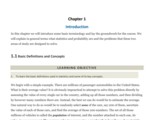Conditional Remix & Share Permitted
CC BY-NC-SA
Rating
0.0 stars

This book is meant to be a textbook for a standard one-semester introductory statistics course for general education students. Our motivation for writing it is twofold: 1.) to provide a low-cost alternative to many existing popular textbooks on the market; and 2.) to provide a quality textbook on the subject with a focus on the core material of the course in a balanced presentation.

Chapter 1: Introduction
Chapter 2: Descriptive Statistics
Chapter 3: Basic Concepts of Probability
Chapter 4: Discrete Random Variables
Chapter 5: Continuous Random Variables
Chapter 6: Sampling Distributions
Chapter 7: Estimation
Chapter 8: Testing Hypotheses
Chapter 9: Two-Sample Problems
Chapter 10: Correlation and Regression
Chapter 11: Chi-Square Tests and F-Tests

Access also available here: https://resources.saylor.org/wwwresources/archived/site/textbooks/Introductory%20Statistics.pdf

Subject:
Mathematics
Statistics and Probability
Material Type:
Textbook
Provider:
The Saylor Foundation
Provider Set:
Saylor Textbooks
Author:
Douglas Shafer
Zhiyi Zhang
04/24/2019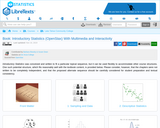Conditional Remix & Share Permitted
CC BY-NC-SA
Rating
0.0 stars

Introductory Statistics was conceived and written to fit a particular topical sequence, but it can be used flexibly to accommodate other course structures. One such potential structure, which fits reasonably well with the textbook content, is provided below. Please consider, however, that the chapters were not written to be completely independent, and that the proposed alternate sequence should be carefully considered for student preparation and textual consistency.

1: Sampling and Data
Included in this chapter are the basic ideas and words of probability and statistics. You will soon understand that statistics and probability work together. You will also learn how data are gathered and what "good" data can be distinguished from "bad."
1.1: Introduction
1.2: Definitions of Statistics, Probability, and Key Terms
1.3: Data, Sampling, and Variation in Data and Sampling
1.4: Frequency, Frequency Tables, and Levels of Measurement
1.5: Experimental Design and Ethics
1.6: Data Collection Experiment (Worksheet)
1.7: Sampling Experiment (Worksheet)
1.E: Sampling and Data (Exercises)

2: Descriptive Statistics
In this chapter, you will study numerical and graphical ways to describe and display your data. This area of statistics is called "Descriptive Statistics." You will learn how to calculate, and even more importantly, how to interpret these measurements and graphs.
2.1: Prelude to Descriptive Statistics
2.2: Stem-and-Leaf Graphs (Stemplots), Line Graphs, and Bar Graphs
2.3: Histograms, Frequency Polygons, and Time Series Graphs
2.4: Measures of the Location of the Data
2.4E: Measures of the Location of the Data (Exercises)
2.5: Box Plots
2.6: Measures of the Center of the Data
2.7: Skewness and the Mean, Median, and Mode
2.8: Measures of the Spread of the Data
2.9: Descriptive Statistics (Worksheet)
2.E: Descriptive Statistics (Exercises)

3: Probability Topics
Probability theory is concerned with probability, the analysis of random phenomena. The central objects of probability theory are random variables, stochastic processes, and events: mathematical abstractions of non-deterministic events or measured quantities that may either be single occurrences or evolve over time in an apparently random fashion.
3.1: Introduction
3.2: Terminology
3.3: Independent and Mutually Exclusive Events
3.4: Two Basic Rules of Probability
3.5: Contingency Tables
3.6: Tree and Venn Diagrams
3.7: Probability Topics (Worksheet)
3.E: Probability Topics (Exercises)

4: Discrete Random Variables
4.1: Prelude to Discrete Random Variables
4.2: Probability Distribution Function (PDF) for a Discrete Random Variable
4.3: Mean or Expected Value and Standard Deviation
4.4: Binomial Distribution
4.5: Discrete Distribution (Playing Card Experiment)
4.6: Discrete Distribution (Lucky Dice Experiment)
4.E: Discrete Random Variables (Exercises)

5: Continuous Random Variables
5.1: Introduction
5.2: Continuous Probability Functions
5.3: The Uniform Distribution
5.4: Continuous Distribution (Worksheet)
5.E: Continuous Random Variables (Exercises)
5.E: Exercises

6: The Normal Distribution
In this chapter, you will study the normal distribution, the standard normal distribution, and applications associated with them. The normal distribution has two parameters (two numerical descriptive measures), the mean ( μμ ) and the standard deviation ( σσ ).
6.1: Prelude to The Normal Distribution
6.2: The Standard Normal Distribution
6.1E: The Standard Normal Distribution (Exercises)
6.3: Using the Normal Distribution
6.4: Normal Distribution - Lap Times (Worksheet)
6.5: Normal Distribution - Pinkie Length (Worksheet)
6.E: The Normal Distribution (Exercises)

7: The Central Limit Theorem
In a population whose distribution may be known or unknown, if the size (n) of samples is sufficiently large, the distribution of the sample means will be approximately normal. The mean of the sample means will equal the population mean. The standard deviation of the distribution of the sample means, called the standard error of the mean, is equal to the population standard deviation divided by the square root of the sample size (n).
7.1: Prelude to the Central Limit Theorem
7.2: The Central Limit Theorem for Sample Means (Averages)
7.2E: The Central Limit Theorem for Sample Means (Exercises)
7.3: The Central Limit Theorem for Sums
7.4: Using the Central Limit Theorem
7.4E: Using the Central Limit Theorem (Exercises)
7.5: Central Limit Theorem - Pocket Change (Worksheet)
7.6: Central Limit Theorem - Cookie Recipes (Worksheet)
7.E: The Central Limit Theorem (Exercises)

8: Confidence Intervals
In this chapter, you will learn to construct and interpret confidence intervals. You will also learn a new distribution, the Student's-t, and how it is used with these intervals. Throughout the chapter, it is important to keep in mind that the confidence interval is a random variable. It is the population parameter that is fixed.
8.1: Prelude to Confidence Intervals
8.2: A Single Population Mean using the Normal Distribution
8.2E: A Single Population Mean using the Normal Distribution (Exercises)
8.3: A Single Population Mean using the Student t-Distribution
8.4: A Population Proportion
8.5: Confidence Interval - Home Costs (Worksheet)
8.6: Confidence Interval -Place of Birth (Worksheet)
8.7: Confidence Interval -Women's Heights (Worksheet)
8.E: Confidence Intervals (Exercises)
8.S: Confidence Intervals (Summary)

9: Hypothesis Testing with One Sample
9.1: Prelude to Hypothesis Testing
9.2: Null and Alternative Hypotheses
9.1E: Null and Alternative Hypotheses (Exercises)
9.3: Outcomes and the Type I and Type II Errors
9.2E: Outcomes and the Type I and Type II Errors (Exercises)
9.4: Distribution Needed for Hypothesis Testing
9.3E: Distribution Needed for Hypothesis Testing (Exercises)
9.5: Rare Events, the Sample, Decision and Conclusion
9.4E: Rare Events, the Sample, Decision and Conclusion (Exercises)
9.6: Additional Information and Full Hypothesis Test Examples
9.7: Hypothesis Testing of a Single Mean and Single Proportion (Worksheet)
9.E: Hypothesis Testing with One Sample (Exercises)

10: Hypothesis Testing with Two Samples
You have learned to conduct hypothesis tests on single means and single proportions. You will expand upon that in this chapter. You will compare two means or two proportions to each other. To compare two means or two proportions, you work with two groups. The groups are classified either as independent or matched pairs.
10.1: Prelude to Hypothesis Testing with Two Samples
10.2: Two Population Means with Unknown Standard Deviations
10.3: Two Population Means with Known Standard Deviations
10.4: Comparing Two Independent Population Proportions
10.5: Matched or Paired Samples
10.6: Hypothesis Testing for Two Means and Two Proportions (Worksheet)
10.E: Hypothesis Testing with Two Samples (Exercises)

11: The Chi-Square Distribution
11.1: Prelude to The Chi-Square Distribution
11.2: Facts About the Chi-Square Distribution
11.3: Goodness-of-Fit Test
11.4: Test of Independence
11.5: Test for Homogeneity
11.6: Comparison of the Chi-Square Tests
11.7: Test of a Single Variance (Not Included in the Course)
11.8: Lab 1: Chi-Square Goodness-of-Fit (Worksheet)
11.9: Lab 2: Chi-Square Test of Independence (Worksheet)
11.E: The Chi-Square Distribution (Exercises)

12: Linear Regression and Correlation
Regression analysis is a statistical process for estimating the relationships among variables and includes many techniques for modeling and analyzing several variables. When the focus is on the relationship between a dependent variable and one or more independent variables.
12.1: Prelude to Linear Regression and Correlation
12.2: Linear Equations
12.2E: Linear Equations (Exercises)
12.3: Scatter Plots
12.3E: Scatter Plots (Exercises)
12.4: The Regression Equation
12.4E: The Regression Equation (Exercise)
12.5: Testing the Significance of the Correlation Coefficient
12.5E: Testing the Significance of the Correlation Coefficient (Exercises)
12.6: Prediction
12.6E: Prediction (Exercises)
12.7: Outliers
12.7E: Outliers (Exercises)
12.8: Regression - Distance from School (Worksheet)
12.9: Regression - Textbook Cost (Worksheet)
12.10: Regression - Fuel Efficiency (Worksheet)
12.E: Linear Regression and Correlation (Exercises)

13: F Distribution and One-Way ANOVA
For hypothesis tests comparing averages between more than two groups, statisticians have developed a method called "Analysis of Variance" (abbreviated ANOVA). In this chapter, you will study the simplest form of ANOVA called single factor or one-way ANOVA. You will also study the FF distribution, used for one-way ANOVA, and the test of two variances. This is just a very brief overview of one-way ANOVA.
13.1: Prelude to F Distribution and One-Way ANOVA
13.2: One-Way ANOVA
13.3: The F Distribution and the F-Ratio
13.4: Facts About the F Distribution
13.5: Lab: One-Way ANOVA
13.E: F Distribution and One-Way ANOVA (Exercises)

Subject:
Mathematics
Statistics and Probability
Material Type:
Full Course
Author:
Barbara Illowsky
Susan Dean
04/29/2020Conditional Remix & Share Permitted
CC BY-SA
Rating
0.0 stars

We hope readers will take away three ideas from this book in addition to forming a foundation of statistical thinking and methods.

(1) Statistics is an applied field with a wide range of practical applications.

(2) You don't have to be a math guru to learn from interesting, real data.

(3) Data are messy, and statistical tools are imperfect. However, when you understand the strengths and weaknesses of these tools, you can use them to learn interesting things about the world.

Reviews available here: https://open.umn.edu/opentextbooks/textbooks/introductory-statistics-with-randomization-and-simulation-first-edition

Subject:
Mathematics
Statistics and Probability
Material Type:
Textbook
Provider:
OpenIntro
Author:
Christopher Barr
David Diez
Mine Çetinkaya-Runde
04/24/2019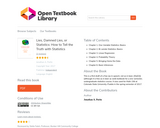Conditional Remix & Share Permitted
CC BY-SA
Rating
0.0 stars

Textbook for a one-semester, undergraduate statistics course. It was used for Math 156 at Colorado State University–Pueblo in the spring semester of 2017.

Chapter 1. One-Variable Statistics: Basics
Chapter 2. Bi-variate Statistics: Basics
Chapter 3. Linear Regression
Chapter 4. Probability Theory
Chapter 5. Bringing Home the Data
Chapter 6. Basic Inferences

Subject:
Mathematics
Statistics and Probability
Material Type:
Textbook
Author:
Jonathan A. Poritz
06/23/2020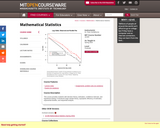Conditional Remix & Share Permitted
CC BY-NC-SA
Rating
0.0 stars

This course provides students with decision theory, estimation, confidence intervals, and hypothesis testing. It introduces large sample theory, asymptotic efficiency of estimates, exponential families, and sequential analysis.

Subject:
Mathematics
Statistics and Probability
Material Type:
Full Course
Provider:
M.I.T.
Provider Set:
M.I.T. OpenCourseWare
Author:
Peter Kempthorne
01/01/2016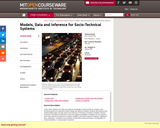Conditional Remix & Share Permitted
CC BY-NC-SA
Rating
0.0 stars

In this class, students use data and systems knowledge to build models of complex socio-technical systems for improved system design and decision-making. Students will enhance their model-building skills, through review and extension of functions of random variables, Poisson processes, and Markov processes; move from applied probability to statistics via Chi-squared t and f tests, derived as functions of random variables; and review classical statistics, hypothesis tests, regression, correlation and causation, simple data mining techniques, and Bayesian vs. classical statistics. A class project is required.

Subject:
Mathematics
Statistics and Probability
Material Type:
Full Course
Provider:
M.I.T.
Provider Set:
M.I.T. OpenCourseWare
Author:
Frey, Daniel
01/01/2007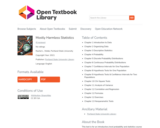Conditional Remix & Share Permitted
CC BY-SA
Rating
0.0 stars

This text is for an introductory level probability and statistics course with an intermediate algebra prerequisite. The focus of the text follows the American Statistical Association’s Guidelines for Assessment and Instruction in Statistics Education (GAISE). Software examples provided for Microsoft Excel, TI-84 & TI-89 calculators. A formula packet and pdf version of the text are available on the website http://mostlyharmlessstatistics.com. Students new to probability and statistics are sure to benefit from this fully ADA accessible and relevant textbook. The examples resonate with everyday life, the text is approachable, and has a conversational tone to provide an inclusive and easy to read format for students.

able of Contents
Chapter 1 Introduction to Data
Chapter 2 Organizing Data
Chapter 3 Descriptive Statistics
Chapter 4 Probability
Chapter 5 Discrete Probability Distributions
Chapter 6 Continuous Probability Distributions
Chapter 7 Confidence Intervals for One Population
Chapter 8 Hypothesis Tests for One Population
Chapter 9 Hypothesis Tests & Confidence Intervals for Two Populations
Chapter 10 Chi-Square Tests
Chapter 11 Analysis of Variance
Chapter 12 Correlation and Regression
Chapter 12 Formulas
Chapter 12 Exercises
Chapter 13 Nonparametric Tests

Subject:
Mathematics
Statistics and Probability
Material Type:
Textbook
Author:
Portland State University
Rachel L. Webb
10/14/2021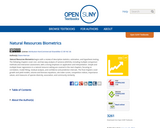Conditional Remix & Share Permitted
CC BY-NC
Rating
0.0 stars

Natural Resources Biometrics begins with a review of descriptive statistics, estimation, and hypothesis testing. The following chapters cover one- and two-way analysis of variance (ANOVA), including multiple comparison methods and interaction assessment, with a strong emphasis on application and interpretation. Simple and multiple linear regressions in a natural resource setting are covered in the next chapters, focusing on correlation, model fitting, residual analysis, and confidence and prediction intervals. The final chapters cover growth and yield models, volume and biomass equations, site index curves, competition indices, importance values, and measures of species diversity, association, and community similarity.

Subject:
Mathematics
Statistics and Probability
Material Type:
Textbook
Provider:
State University of New York
Provider Set:
OpenSUNY Textbooks
Author:
Diane Kiernan
01/16/2014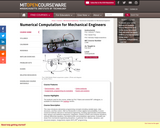Conditional Remix & Share Permitted
CC BY-NC-SA
Rating
0.0 stars

This class introduces elementary programming concepts including variable types, data structures, and flow control. After an introduction to linear algebra and probability, it covers numerical methods relevant to mechanical engineering, including approximation (interpolation, least squares and statistical regression), integration, solution of linear and nonlinear equations, ordinary differential equations, and deterministic and probabilistic approaches. Examples are drawn from mechanical engineering disciplines, in particular from robotics, dynamics, and structural analysis. Assignments require MATLAB programming.

Subject:
Applied Science
Calculus
Engineering
Information Science
Mathematics
Statistics and Probability
Material Type:
Full Course
Provider:
M.I.T.
Provider Set:
M.I.T. OpenCourseWare
Author:
Anthony Patera
Daniel Frey